# Adsorption and Applications Surface Chemistry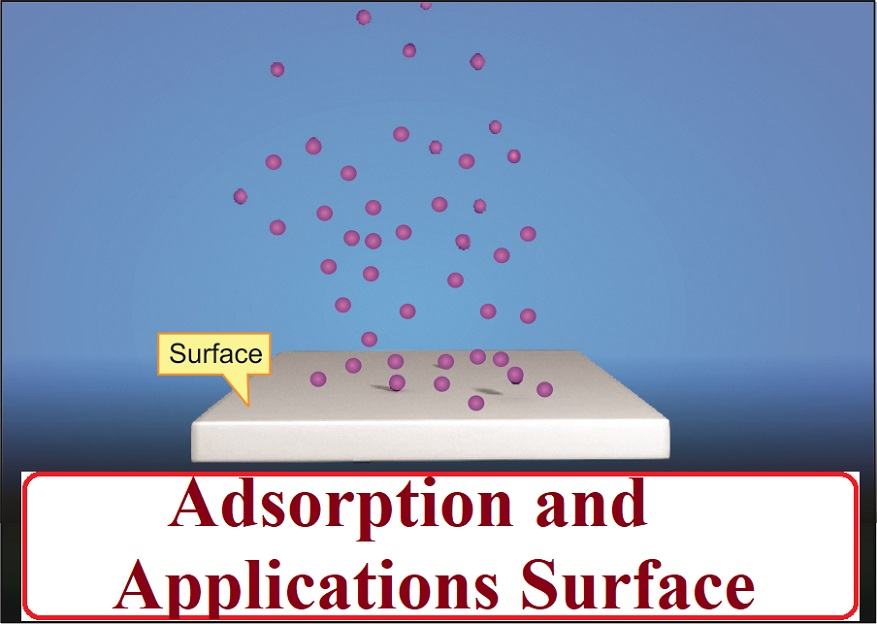Surface Chemistry is the branch of chemistry that deals with the study of the phenomenon occurring at the surface than bulk.

Adsorption: The accumulation of molecular species at the surface rather than in the bulk of a solid or liquid is termed as adsorption.

The commonly used adsorbents are charcoal, silica gel, alumina gel, clay, colloids, metals in a finely divided state, etc.

1. Powdered charcoal absorbs gases like H2, O2, CO, Cl2, NH3, SO2
2. Silica gel absorbs moisture
3. Animal charcoal adsorbs colouring material from sugar solutions.

Desorption: The removal of the adsorbed substance from a surface is called desorption.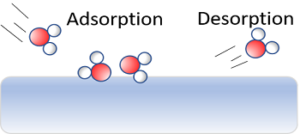Sorption: If adsorption and absorption occur simultaneously, the process is called sorption.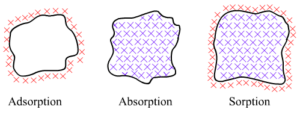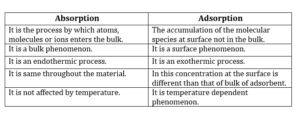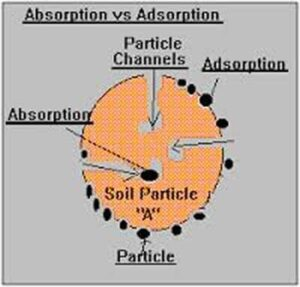The surface particles of the adsorbent are not in the same environment as the particles inside the bulk (inner part). Inside the adsorbent, all the forces are mutually balanced. But at the surface, there is always some unbalanced or residual forces. These forces of the adsorbent are responsible for adsorption.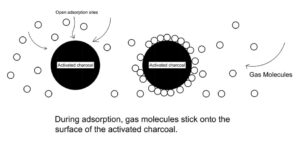During adsorption, there is always a decrease in residual force i.e., there is a decrease in surface energy, which appears as heat. Therefore, adsorption is an exothermic process i.e. ΔH = – ve. Also, the movement of the particles is restricted in this process. Therefore ΔS = – ve According to Gibbs Helmholtz equation:
ΔG = ΔH – TΔS,  or    ΔG = (–ΔH ) – T(–ΔS) for adsorption to occur.
ΔG must be negative which is possible only when ΔH >TΔS.

(i) Physical adsorption: When the particles of adsorbate are held to the surface of the adsorbent by weak van der Waals forces.
Characteristics of physical adsorption: Lack of specificity, low enthalpy of adsorption, reversible in nature, no activation energy required, decrease with an increase in temperature.

(ii) Chemical adsorption: When the molecules of adsorbate are held to the surface of the adsorbent by strong chemical forces.
Characteristics of chemical adsorption: Highly specific in nature, high enthalpy of adsorption, Irreversible in nature, initially it increases with an increase in temperature as it needs activation energy, very slow.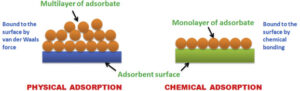Freundlich Adsorption Isotherm: The variation in the amount of gas adsorbed by the adsorbent with pressure at constant temperature can be expressed by means of a curve termed as adsorption isotherm. (It is a plot/curve between the extent of adsorption (x/m) and pressure P at constant T).
The relationship can be expressed by the following equation:
x/m = k.P1/n (where n > 1)
where x is the mass of the gas adsorbed, m is the mass of the adsorbent, k and n are constants that depend on the nature of the adsorbent and the gas at a particular temperature.
The relationship can be expressed by the following equation:
x/m = k.P1/n (where n > 1)
where x is the mass of the gas adsorbed, m is the mass of the adsorbent, k and n are constants that depend on the nature of the adsorbent and the gas at a particular temperature.
The above relationship can be represented in the form of a graph as follows:
The plot of x/ m  vs log P is a straight line with slope and intercept on Y-axis = log k.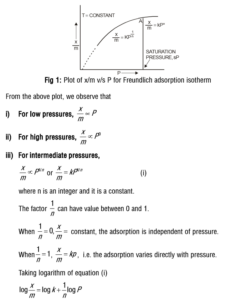Limitations of Freundlich Isotherm:
(i) It is unable to explain the deviation from linearity at high pressure.
(ii) It is applicable only for physical adsorption as it considers the multimolecular layer of adsorption.
(iii) It doesn’t consider the role of the surface area of adsorbent in the process of adsorption The Freundlich isotherm was modified by Langmuir in 1916.

(i) Production of high vacuum: For the complete evacuation of a vessel, activated charcoal is used.
(ii) Gas masks: The poisonous gases in coal mines can be removed by using gas masks containing activated charcoal.
(iii) Control of humidity: Silica and aluminium gels are used as adsorbents for removing moisture and controlling humidity.
(iv) Animal charcoal is used for the purification of cane sugar solution.
(v) Adsorption finds application in heterogeneous catalysis.
(vi) A mixture of noble gases can be separated by adsorption on coconut charcoal at different temperatures.
(vii) In curing diseases: A number of drugs are used to kill germs by getting adsorbed on them.
(viii) In the froth floatation process for the purification of sulphide ores in metallurgy.
(ix) Adsorption indicators like eosin, fluorescein, etc. are used in volumetric analysis.
(x) Chromatographic analysis for the separation of a mixture is based on adsorption.

# Kore PVC 16-30 Kg Home Gym Set with One 3 Ft Curl and One Pair Dumbbell Rods with Gym Accessories